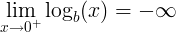# Logarithm rules

## Logarithm Rules and Properties

Rule name Rule
Logarithm product rule

logb(x ∙ y) = logb(x) + logb(y)

Logarithm quotient rule

logb(x / y) = logb(x) - logb(y)

Logarithm power rule

logb(x y) = y ∙ logb(x)

Logarithm base switch rule

logb(c) = 1 / logc(b)

Logarithm base change rule

logb(x) = logc(x) / logc(b)

Derivative of logarithm

f (x) = logb(x) f ' (x) = 1 / ( x ln(b) )

Integral of logarithm

logb(x) dx = x ∙ ( logb(x) - 1 / ln(b) ) + C

Logarithm of 0

logb(0) is undefinedLogarithm of 1

logb(1) = 0

Logarithm of the base

logb(b) = 1

Logarithm of infinity

lim logb(x) = ∞, when x→∞

## Logarithm product rule

The logarithm of a multiplication of x and y is the sum of logarithm of x and logarithm of y.

logb(x ∙ y) = logb(x) + logb(y)

#### For example:

logb(37) = logb(3) + logb(7)

The product rule can be used for fast multiplication calculation using addition operation.

The product of x multiplied by y is the inverse logarithm of the sum of logb(x) and logb(y):

x ∙ y = log-1(logb(x) + logb(y))

## Logarithm quotient rule

The logarithm of a division of x and y is the difference of logarithm of x and logarithm of y.

logb(x / y) = logb(x) - logb(y)

#### For example:

logb(3 / 7) = logb(3) - logb(7)

The quotient rule can be used for fast division calculation using subtraction operation.

The quotient of x divided by y is the inverse logarithm of the subtraction of logb(x) and logb(y):

x / y = log-1(logb(x) - logb(y))

## Logarithm power rule

The logarithm of the exponent of x raised to the power of y, is y times the logarithm of x.

logb(x y) = y ∙ logb(x)

#### For example:

logb(28) = 8logb(2)

The power rule can be used for fast exponent calculation using multiplication operation.

The exponent of x raised to the power of y is equal to the inverse logarithm of the multiplication of y and logb(x):

x y = log-1(y ∙ logb(x))

## Logarithm base switch

The base b logarithm of c is 1 divided by the base c logarithm of b.

logb(c) = 1 / logc(b)

#### For example:

log2(8) = 1 / log8(2)

## Logarithm base change

The base b logarithm of x is base c logarithm of x divided by the base c logarithm of b.

logb(x) = logc(x) / logc(b)

## Logarithm of 0

The base b logarithm of zero is undefined:

logb(0) is undefined

The limit near 0 is minus infinity:## Logarithm of 1

The base b logarithm of one is zero:

logb(1) = 0

log2(1) = 0

## Logarithm of the base

The base b logarithm of b is one:

logb(b) = 1

log2(2) = 1

## Logarithm derivative

When

f (x) = logb(x)

Then the derivative of f(x):

f ' (x) = 1 / ( x ln(b) )

#### For example:

When

f (x) = log2(x)

Then the derivative of f(x):

f ' (x) = 1 / ( x ln(2) )

## Logarithm integral

The integral of logarithm of x:

logb(x) dx = x ∙ ( logb(x) - 1 / ln(b) ) + C

#### For example:

log2(x) dx = x ∙ ( log2(x) - 1 / ln(2) ) + C

## Logarithm approximation

log2(x) ≈ n + (x/2n - 1) ,

Logarithm of zero »

Currently, we have around 929 calculators and conversion tables to help you "do the math" quickly in areas such as:

And we are still developing more. Our goal is to become the one-stop, go-to site for people who need to make quick calculations or who need to find quick answer for basic conversions.

Additionally, we believe the internet should be a source of free information. Therefore, all of our tools and services are completely free, with no registration required. We coded and developed each calculator individually and put each one through strict, comprehensive testing. However, please inform us if you notice even the slightest error – your input is extremely valuable to us. While most calculators on Justfreetools.com are designed to be universally applicable for worldwide usage, some are for specific countries only.